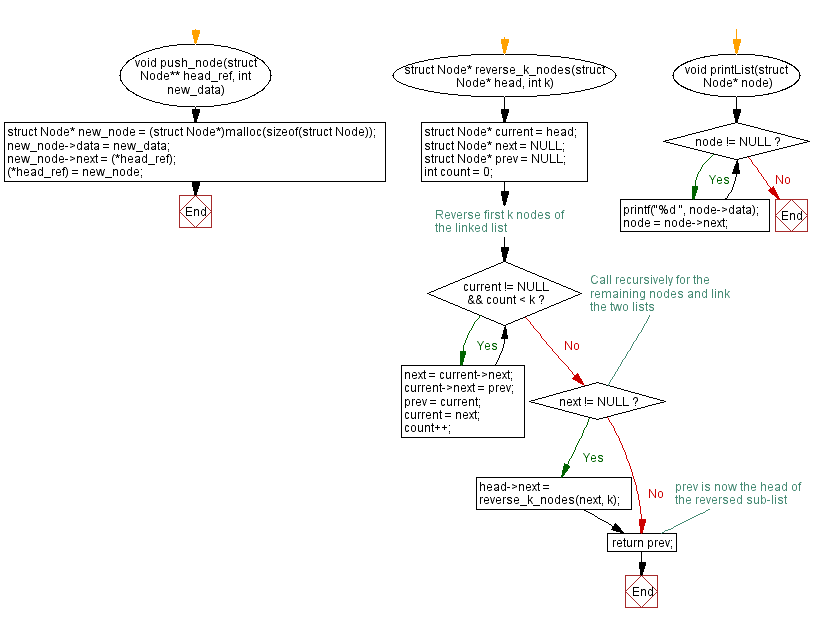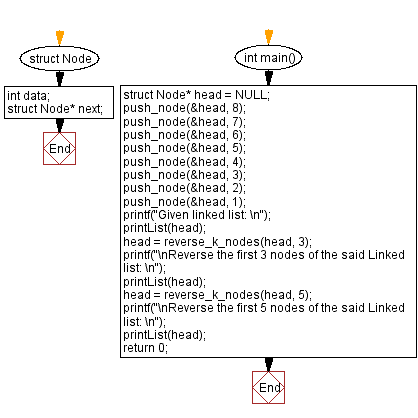﻿ C Program: Reverse a singly linked list in blocks of size k

# C Exercises: Reverse a singly linked list in blocks of size k

## C Singly Linked List : Exercise-36 with Solution

Write a C program to reverse a singly linked list starting at the first position in blocks of size k.

Sample Solution:

C Code:

``````#include<stdio.h>
#include <stdlib.h>

struct Node {
int data;
struct Node* next;
};

void push_node(struct Node** head_ref, int new_data) {
struct Node* new_node = (struct Node*)malloc(sizeof(struct Node));
new_node->data = new_data;
}

struct Node* reverse_k_nodes(struct Node* head, int k) {
struct Node* next = NULL;
struct Node* prev = NULL;
int count = 0;

/* Reverse first k nodes of the linked list */
while (current != NULL && count < k) {
next = current->next;
current->next = prev;
prev = current;
current = next;
count++;
}

/* Call recursively for the remaining nodes and link the two lists */
if (next != NULL) {
}

/* prev is now the head of the reversed sub-list */
return prev;
}

void printList(struct Node* node) {
while (node != NULL) {
printf("%d ", node->data);
node = node->next;
}
}

int main() {

printf("\nReverse the first 3 nodes of the said Linked list: \n");
printf("\nReverse the first 5 nodes of the said Linked list: \n");
return 0;
}
```
```

Sample Output:

```Given linked list:
1 2 3 4 5 6 7 8
Reverse the first 3 nodes of the said Linked list:
3 2 1 6 5 4 8 7
Reverse the first 5 nodes of the said Linked list:
5 6 1 2 3 7 8 4
```

Flowchart :C Programming Code Editor:

What is the difficulty level of this exercise?

﻿